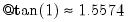Command Reference : Operator and Function Reference : Trigonometric Functions

Trigonometric Functions
When applied to a series, all of the trigonometric functions operate on every observation in the current sample and return a value for every observation. Where relevant, the input and results should/will be expressed in radians. All results are real valued—complex values will return NAs.

 Function Name Examples/Description @acos(x) arc cosine (real results in radians)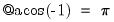@asin(x) arc sine (real results in radians)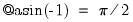@atan(x) arc tangent (results in radians)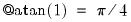@cos(x) cosine (argument in radians)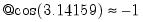@sin(x) sine (argument in radians)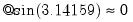@tan(x) tangent (argument in radians)